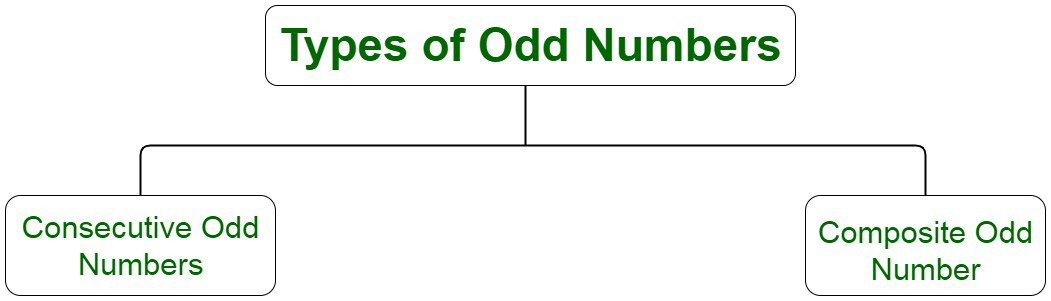GFG App
Open AppBrowser
Continue

# Find the mean of the first 10 odd natural numbers

Natural numbers are counting numbers beginning with 1. They include all the positive integer numbers beginning with 1 up till infinity. Some of the examples of natural numbers are 0, 5, 13, 32, 45, 57, 69, etc.

Odd numbers are the integers that are not divisible by 2. These types of numbers cannot be divided by 2 evenly, that is, these numbers cannot be segregated into two equal parts. Some of the examples of odd numbers are 1,3,5 etc. Odd numbers may be negative or positive in nature. These numbers leave a remainder on division with 2. These numbers are completely opposite in nature to even numbers.

### Types of Odd Numbers

There are majorly two types of odd numbers:

Consecutive odd numbers

For any odd natural number n, the numbers n and n + 2 fall under the category of consecutive odd numbers. All the consecutive odd numbers have a difference of 2 between them. For example 3 and 5, 7 and 11. There are infinite such pairs.

Composite odd numbers

Composite odd numbers are made up of several parts or elements. Odd integers which are not prime are odd composite numbers. The composite odd numbers from 1 to 20 are 9 and 15.Mean

Mean is one of the statistical concepts which is used to calculate the average or a central value of a given set of integers. It gives information about the central tendency of the data. The central tendency of the data is a statistical measure that uses a single value to gather information about the entire set of data distribution. It provides a description of the entire data set.

Mean is equivalent to the sum of all observations to the total number of observations. Mean is also called the arithmetic mean or average. Let us assume a data set to be given by, X = x1, x2,…, xn. The mean is denoted by x̄ which is the mean of given n values.

According to the definition, we have,

X̄ = (Sum of values ÷ Number of values)Some of the examples of mean in our real life are:

• Mean of marks scored by students in a test.
• Mean of runs scored by a cricketer in a one day match.

Computing Mean

Mean of the data set can be calculated by computing the average of the numbers. In the case of negative numbers, the summation of data values is calculated and then divided by the number of negative integers.

For instance, if we have five random integer values, such as -1, 0, 6, 9, 1, we can compute mean by calculating the average of these numbers, which is given by,

Average = Sum / Number of such values

Sum = -1 + 0 + 6 + 5 + 1

= 15

Number of values = 5

Average = 15/5 = 3

Therefore, the mean of these values is equivalent to 3.

### Question: Find the mean of the first 10 odd natural numbers

Natural numbers are the counting numbers beginning with 1. The first 10 odd counting numbers are 1, 3, 5, 7, 9, 11, 13, 15, 17, 19.

On computing the summation of these natural numbers, we have,

Sum of numbers = 1 + 3 + 5 + 7 + 9 + 11 + 13 + 15 + 17 + 19

= 100

Count of numbers = 10

Mean of numbers = Sum of numbers / Count of numbers

= 100 / 10

= 10

### Sample Questions

Question 1: How can it be determined that a number is even or odd?

A number that is divisible by 2 is known as an even number. An even number leaves a remainder of 0 on the division of 2. For example, 2, 4, 6, etc are even numbers. A number not divisible by 2 is known as an odd number. For example, 1,3, and 5 are odd numbers. The unit digits of the number can be checked in order to make sure that the number is even or odd. If unit place carries an even number, that means the whole number is even else it’s odd in nature.

Question 2: State whether zero is an odd number or not.

No, zero on division with the integer value of 2, leaves a remainder of 0. The quotient of the division is 0. Therefore, zero is an even number.

0 ÷ 2 = 0

Question 3: The mean of 3 odd numbers is 10. Two of them are 6 and 9. Calculate the third number.

Let the third number be x.

Now, we have,

Sum of numbers = 6 + 9 + x

Mean of numbers = (15+x)/3

Substituting the values, we have,

10 × 3 = (15 + x)

30 = 15 + x

x = 15

Therefore, the third number is 15.

Question 4: Calculate mean of the number n, n + 4 in terms of n.

Mean = (n + n + 4)/2

= (2n + 4)/2

= n + 2

Question 5: Calculate mean of the first five odd natural numbers.